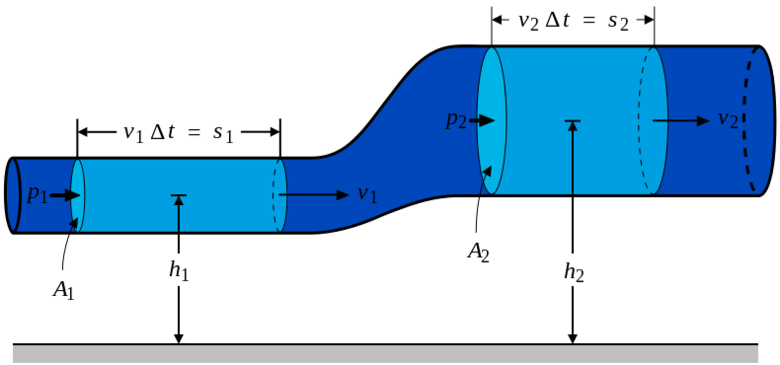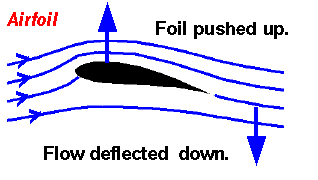# Bernoulli's equation

Bernoulli's equation expresses conservation of energy for flowing fluids (specifically incompressible fluids), such as water. It shows the equivalence of the overall energy for a given volume of a fluid as it moves. The equation used relates the energy of the fluid in terms of its elevation, pressure, and velocity and relies on the principles outlined by the law of conservation of energy. This equation can be expressed as:

or in it's conservation of energy form as:

where the left side is some fluid at the first position and the right side is the same fluid having moved to the second position. Each term represents the energy per unit volume of the fluid. The first term represents the pressure energy, the second represents the kinetic energy, and the third represents gravitational potential energy. The variables are defined as:

• is the fluid pressure
• is the fluid density
• is the fluid velocity
• is the acceleration due to gravity
• is the elevation of the fluid above a fixed reference pointFigure 1. A diagram of a pipe, illustrating the different aspects of Bernoulli's equation. Here, the pressure, elevation, and velocity of the fluid within the pipe changes from position 1 and position 2. However, when the values for elevation, pressure, and velocity at both locations are input into the equation above, they result in an equality as the overall energy must be conserved.

## Use in the Energy Sector

It is important to note that by rearranging components of this expression, certain important values can be expressed. For example, Bernoulli's equation is important for hydropower and the above equation can be transformed to represent hydraulic head by dividing by the fluid density and the acceleration due to gravity. The expression for hydraulic head is:

This equation can be used to calculate the hydraulic head difference across a hydroelectric dam as well as the head losses, due to friction, that determine the effective head across a dam.

Additionally, Bernoulli's equation is useful with respect to wind power as it is used to relate the velocity of the flow of air to the pressure difference across the turbine. Although useful in determining these pressures and velocities, it cannot be applied to air passing through the wind turbine itself, rather it can only be used to investigate the air flow on either side. The ability to look at how air flows around turbines is useful in determining how the turbines will operate and how much power can be generated.

Finally, Bernoulli's equation is used in Compressed Air Energy Storage or CAES as air is compressed with an air compressor, pressurizing it and pushing it below ground into a storage area. This pressurized air is then used to turn a turbine, and Bernoulli's principle can describe the speed and pressure of the air as it flows to the turbine.

## Examples

Bernoulli's equation can be applied in a number of different situations, but in terms of energy it finds use in determining the energy available in hydroelectricity generation facilities. It can also be used in other fluid mechanics problems, such as explaining how the shape of a plane wing produces lift and why fire hydrants spray water high in the air when they are tested.

### Energy from a Hydropower Station

Moving water has energy in the form of kinetic energy, while water at high elevations has potential energy. Water that is under pressure also has a type of pressure-related energy, and all three types are related by Bernoulli's equation and used in determining how much energy one can obtain from water in a hydropower station.

In a hydroelectric dam, water from a hydroelectric reservoir moves down into the penstocks. These penstocks are effectively large tubes that have a turbine at the end of them. Water flows through this tube at a given speed, and exits at the same speed, provided that the diameter of the tube does not change. If the diameter of the tube does change, the Bernoulli effect comes into play and the velocity of the water increases as the diameter of the tube decreases, leading to a decrease in pressure.

After moving through the turbine and transferring energy to it to generate electricity, water exits at the same speed but the water pressure has changed significantly. Although this might seem counter-intuitive, by breaking apart Bernoulli's equation this phenomenon can be explained. The position of the water has clearly changed, dropping to a lower elevation at the turbine compared to the height at which it was held in the reservoir. Thus there must either be a pressure or velocity change to make up for this change in gravitational potential energy. In the case of a facility that uses a reaction turbine, the pressure of the water changes as it moves through the turbine.

In hydroelectric facilities that use an impulse turbine, the kinetic energy of the water does change as water is shot through a nozzle at high velocity and it strikes the turbine, dramatically decreasing its velocity. In these cases, the kinetic energy of the water changes as it moves through the turbine, transferring the kinetic energy of the fluid to the turbine.

### Lift from a WingFigure 1. A diagram of lift from a plane wing. An upward force is created by the difference in pressure.

Bernoulli's equation is useful in a number of different fluid mechanics problems, for more than just liquids. For example, it can explain how an airplane wing creates lift.

The shape of the wing of an airplane works by splitting the air into two sections, above and below the wing. The top of the wing is curved, which means that the air that flows over the top of the wing must flow faster than the air flowing beneath the wing to allow it to reach the same position in the end. As a result of Bernoulli's equation, it is known that if the kinetic energy of the fluid changes, either the pressure or gravitational potential energy must change to ensure energy conservation. In this case, if the speed of the air above the wing increases, the position or pressure of the air must change. Since the front and back of the wing are at the same height, this means position does not change, leaving only the pressure to change. If the velocity of the air flowing over the wing increases, its pressure must decrease in order to conserve energy. Since pressure above the wing has decreased, the pressure underneath the wing is now greater than the pressure on top of the wing. This disparity in pressure creates an upward force known as lift that lifts the plane into the air.

### Testing a Fire Hydrant

When firefighters test fire hydrants, the water shoots up out of the hydrant high into the air. With Bernoulli's equation, this effect is easy to explain. If the pressure of a fluid is decreased, either its position or velocity must increase. In some cases, such as this one, both changes occur. As the water leaves the pipes inside the hydrant, the pressure decreases to atmospheric pressure. The pressure energy of the fluid is converted to velocity, which shoots the water up out of the pipe, and the height of the resulting spray is directly determined by the pressure in the hydrant.

For more information on Bernoulli's equation and fluid dynamics please see hyperphysics.

## For Further Reading

For further information please see the related pages below:

## Authors and Editors

Bethel Afework, Edwin Cey, Jordan Hanania, James Jenden, Amanda Musgrove, Kailyn Stenhouse, Jason Donev
Last updated: June 4, 2018
Get Citation